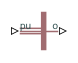# Synchronous Machine Measurement

Per-unit measurement from synchronous machine

•Libraries:
Simscape / Electrical / Electromechanical / Synchronous

## Description

The Synchronous Machine Measurement block outputs a per-unit measurement associated with a connected Synchronous Machine Round Rotor or Synchronous Machine Salient Pole block. The input of the Synchronous Machine Measurement block connects to the `pu` output port of the synchronous machine block.

You set the Output parameter to a per-unit measurement associated with the synchronous machine. Based on the value you select, the Synchronous Machine Measurement block:

• Directly outputs the value of an element in the input signal vector

• Calculates the per-unit measurement by using values of elements in the input signal vector in mathematical expressions

The Synchronous Machine Measurement block outputs a per-unit measurement from the synchronous machine according to the output value expressions in the table. For example, when you set Output to `Stator d-axis voltage`, the block directly outputs the value of the `pu_ed` element in the input signal vector. However, when you set Output to `Reactive power`, the block calculates the value from the `pu_ed`, `pu_eq`, `pu_id`, and `pu_iq` elements.

Output Parameter SettingOutput Value Expression

Field voltage (field circuit base, Efd)

`pu_fd_Efd`

Field current (field circuit base, Ifd)

`pu_fd_Ifd`

Electrical torque

`pu_torque`

Rotor velocity

`pu_velocity`

Stator d-axis voltage

`pu_ed`

Stator q-axis voltage

`pu_eq`

Stator zero-sequence voltage

`pu_e0`

Stator d-axis current

`pu_id`

Stator q-axis current

`pu_iq`

Stator zero-sequence current

`pu_i0`

Apparent power

$\sqrt{pu_P{t}^{2}+pu_Q{t}^{2}}$

Real power

```pu_Pt = (pu_ed*pu_id) + (pu_eq*pu_iq) + 2(pu_e0*pu_i0)```

Reactive power

`pu_Qt = (pu_eq*pu_id) – (pu_ed*pu_iq)`

Terminal voltage

$\sqrt{\left(pu_e{d}^{2}+\text{​}pu_e{q}^{2}\right)}$

Terminal current

$\sqrt{\left(pu_i{d}^{2}+pu_i{q}^{2}\right)}$

`power_factor_angle = atan2(pu_Qt, pu_Pt)`

Power factor

`cos(power_factor_angle)`

`load_angle(rad) = atan2(pu_ed, pu_eq)`

Rotor electrical angle

`y = pi+2*atan(tan((u-pi)/2))`

## Ports

### Input

expand all

Physical signal vector port associated with per-unit measurements from a connected synchronous machine. The vector elements are:

• `pu_fd_Efd`

• `pu_fd_Ifd`

• `pu_torque`

• `pu_velocity`

• `pu_ed`

• `pu_eq`

• `pu_e0`

• `pu_id`

• `pu_iq`

• `pu_i0`

### Output

expand all

Physical signal output port associated with the per-unit measurement.

## Parameters

expand all

Per-unit measurement from synchronous machine. The default value is ```Field voltage (field circuit base, Efd)```.

## Version History

Introduced in R2013b